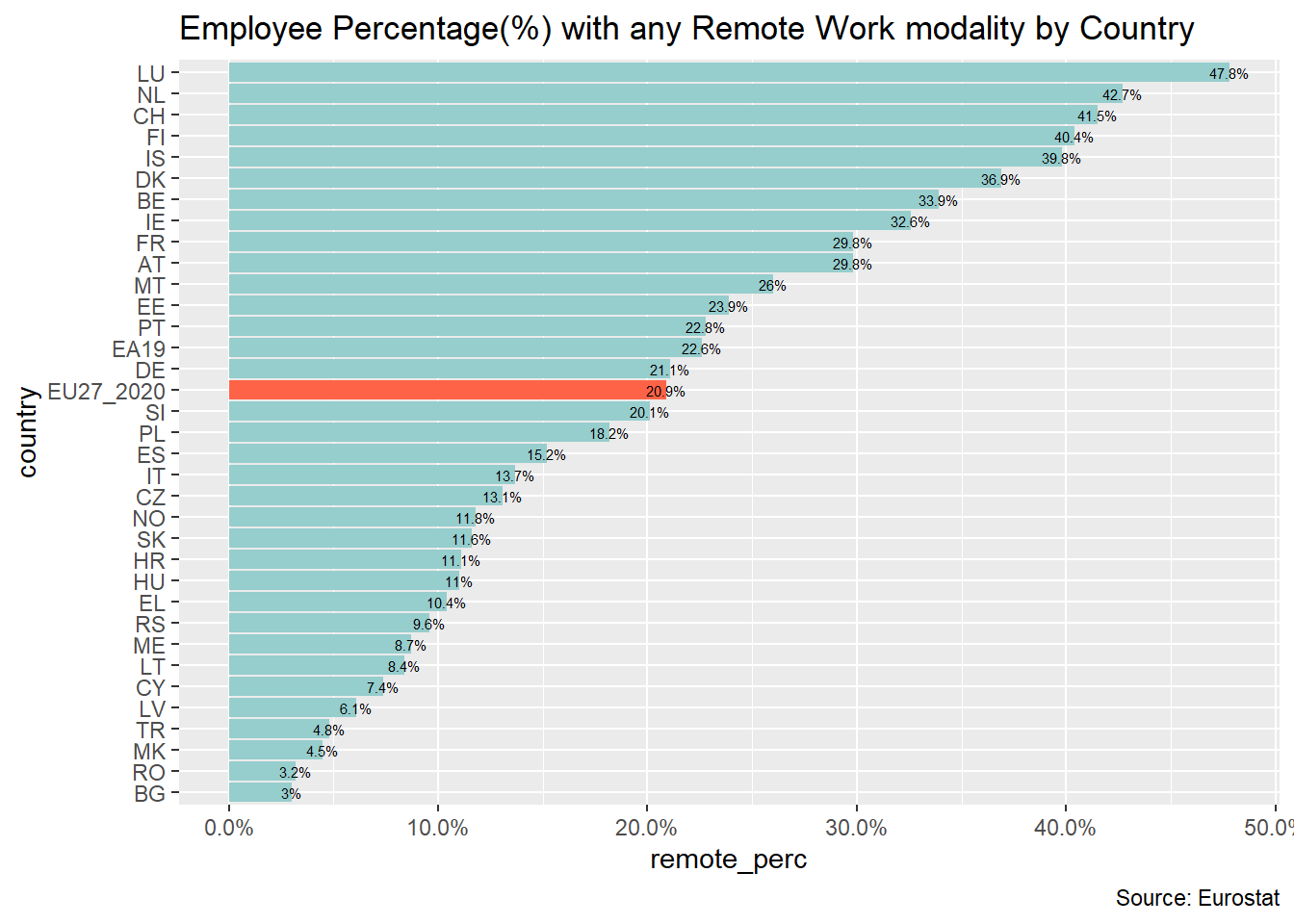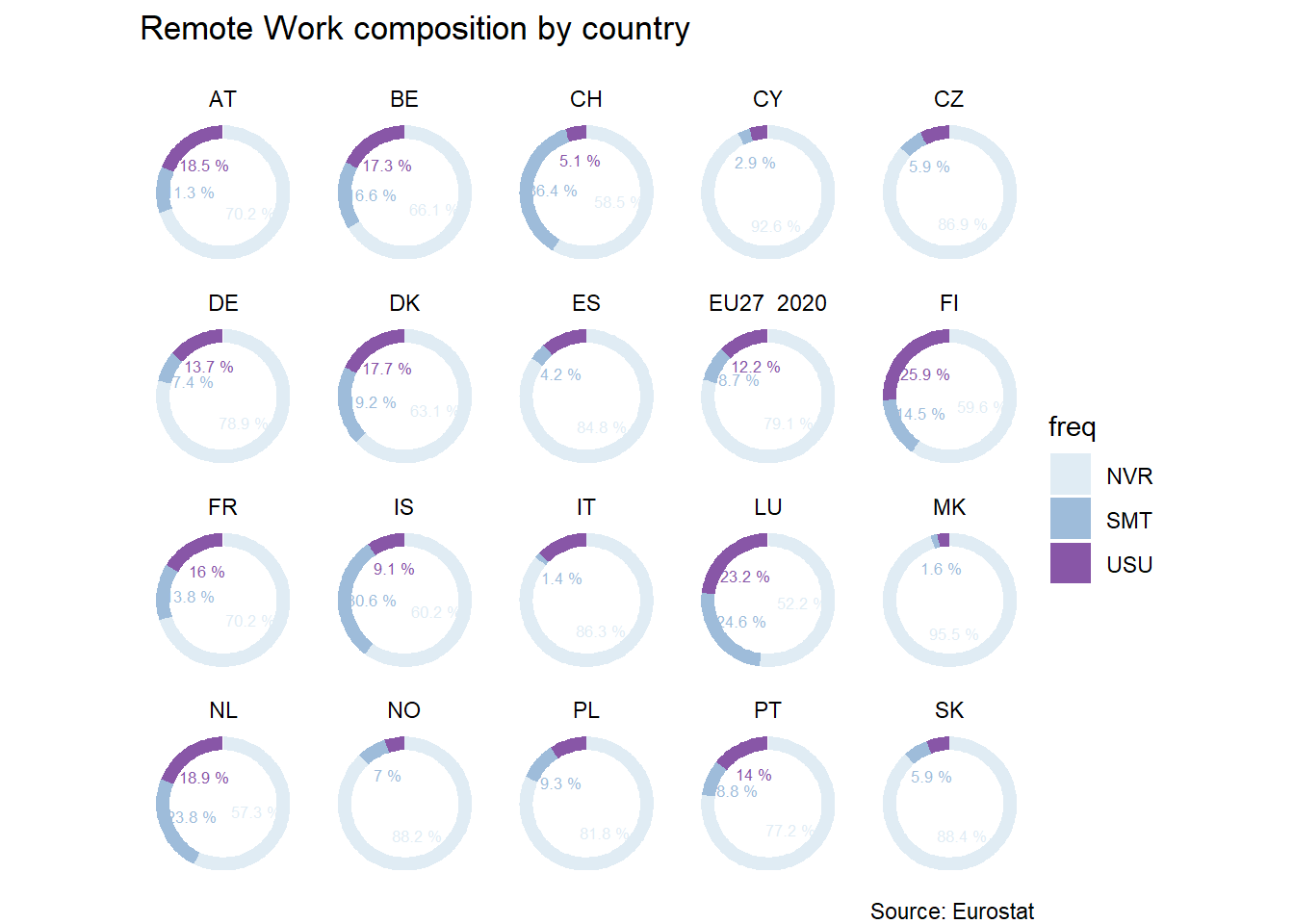Want to share your content on R-bloggers? click here if you have a blog, or here if you don't.As we always do, we are going to connect and download the desired data. In this case, our data source is the Eurostat. We download and read the data file.

```library(tidyverse)

As a first analytical step, we are going to check the TOP 5 countries with employees in any kind of Remote Work / Work From Home (WFH) mode.

```df_european_data <- read_csv(file = 'data.csv',
col_types = cols(sex = col_character())) # Sex columns type

df_european_data_filtered <-df_european_data %>%
filter(freq == "A" & unit == "PC" & wstatus == "EMP" & sex == "T" & age == "Y20-64" & geo != "SE") %>%
select(geo, TIME_PERIOD, OBS_VALUE, frequenc) %>%
rename(remote_perc=OBS_VALUE,
country=geo)

df_european_data_filtered %>%
filter(frequenc == "NVR") %>%
mutate(remote_perc=if_else(frequenc == "NVR",
round(1-remote_perc/100, 3)*100,
round(remote_perc/100, 3)*100)) %>%
select(-frequenc) %>%
arrange(country, TIME_PERIOD) %>%
filter(TIME_PERIOD == "2020") %>%
slice_max(n=6, order_by=remote_perc) %>%
kable()```
country TIME_PERIOD remote_perc
LU 2020 47.8
NL 2020 42.7
CH 2020 41.5
FI 2020 40.4
IS 2020 39.8
DK 2020 36.9

```df_european_data_filtered_bar_plot <- df_european_data_filtered %>%
filter(frequenc == "NVR" & TIME_PERIOD =="2020") %>%
mutate(highlight = if_else(country == "EU27_2020","t","f"),
remote_perc = if_else(frequenc == "NVR",
round(1-remote_perc/100,3),
round(remote_perc/100,3))) %>%
arrange(remote_perc)
df_european_data_filtered_bar_plot %>%
ggplot(aes(country, remote_perc, fill=highlight)) +
geom_bar(stat="identity", position="dodge") +
geom_text(aes(label=paste0(remote_perc*100,"%")), size=2) +
coord_flip() +
scale_x_discrete(limits=df_european_data_filtered_bar_plot\$country) +
scale_fill_manual(values=c( "t"="tomato", "f"="paleturquoise3" ),
guide=FALSE) +
scale_y_continuous(labels=scales::percent) +
labs(title="Employee Percentage(%) with any Remote Work modality by Country",
caption = "Source: Eurostat")```And the TOP 10 with more relative increment?

```df_european_data_filtered %>%
filter(frequenc == "NVR") %>%
mutate( remote_perc = if_else(frequenc == "NVR",
round(1-remote_perc/100,3)*100,
round(remote_perc/100,3)*100)) %>%
select(-frequenc) %>%
arrange(country, TIME_PERIOD) %>%
group_by(country) %>%
mutate(delta = (remote_perc - lag(remote_perc))/lag(remote_perc)*100) %>%
ungroup() %>%
filter(TIME_PERIOD=="2020") %>%
slice_max( n=10,order_by=delta) %>%
kable()```
country TIME_PERIOD remote_perc delta
CY 2020 7.4 196.00000
IT 2020 13.7 191.48936
BG 2020 3.0 172.72727
HU 2020 11.0 139.13043
RO 2020 3.2 128.57143
MT 2020 26.0 122.22222
EL 2020 10.4 100.00000
LT 2020 8.4 86.66667
ES 2020 15.2 80.95238
DE 2020 21.1 63.56589

# 2. Remote worker profile in Europe

## (Usually vs Sometimes vs Never)

A good way to summarize the country labour market profile regarding remote work is to make a donut plot. It’s true that the human brain is not very good at comparing areas, but this plot will allow us to perceive the Remote Work adoption in each modality at a glance.

```list_countries <- c("AT","BE","CH","CY","CZ",
"DE","DK","ES","EU15","EU27_2020","FI",
"FR","IS","IT","LU","MK",
"NL","NO","PL","PT","SK")
ggplot(df_european_data_filtered %>%
filter(TIME_PERIOD=="2020" & (country %in% list_countries )) %>%
group_by(country) %>%
mutate(ymax=cumsum(remote_perc),
ymin=if_else(row_number()==1,0,lag(ymax)),
labelPosition=(ymax+ymin)/2,
label=paste0(remote_perc, " %")) %>%
rename(freq=frequenc),
aes(ymax=ymax, ymin=ymin, xmax=4, xmin=3, fill=freq)) +
geom_rect() +
geom_text(x=1.5,
aes(y=labelPosition, label=label, color=freq),
size=2.2,
check_overlap = T)+
scale_fill_brewer(palette=3) + # donut color
scale_color_brewer(palette=3) + # labs colour
facet_wrap(~country) +
coord_polar(theta="y") +
theme_void() +
xlim(c(-1, 4)) +
labs(title="Remote Work composition by country",
subtitle=" ",
caption = "Source: Eurostat")```## Remote Work temporal evolution

In order to bring more context, we are going to deep into the temporal evolution of each country. We are tracking the employees in each country and year that have been working in some Remote mode. We could see some clusters, with countries with steeper slope than others during the last year in the data reported.

```df_european_data_filtered %>%
filter(frequenc == "NVR" & (country %in% list_countries[list_countries != "CY"])) %>%
mutate( remote_perc = if_else( frequenc == "NVR",round(1-remote_perc/100,3),round(remote_perc/100,3)),) %>%
ggplot(aes(TIME_PERIOD, remote_perc, colour=remote_perc, group=country)) +
geom_line() +
facet_wrap(~country) +
scale_colour_gradient(low = "yellow", high = "red", na.value = NA)+
scale_y_continuous(labels=scales::percent)```## 3. Remote Work ~ Country GDP correlation

As a final thought, the data is pointing out to us that Northern Europe countries are doing more Remote Work. We are expecting that higher GDP countries are more prone to define a Work From Home model or hybrid ones, while the lower GDP ones have more difficulties or are not willing to enhance and embrace change.

The industrial sector composition, kind and size of national companies, politic ideology and government seem such a high impact reasons and probably act as a causality of the heterogenity across the European States.

In the next blog post, we are going to talk and go deeper analyzing the relation between the GDP and the WFH ratios, and discourse about it’s causes and effects.

You can find valuable Remote Work information and community at UbiWork. Work remotely from Spain & Portugal